# Rao-Cramér inequality

(diff) ← Older revision | Latest revision (diff) | Newer revision → (diff)

Cramér–Rao inequality, Fréchet inequality, information inequality

An inequality in mathematical statistics that establishes a lower bound for the risk corresponding to a quadratic loss function in the problem of estimating an unknown parameter.

Suppose that the probability distribution of a random vector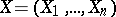with values in the-dimensional Euclidean spaceis defined by a density,,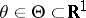. Suppose that a statistic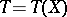such thatis used as an estimator for the unknown scalar parameter, whereis a differentiable function, called the bias of. Then under certain regularity conditions on the family, one of which is that the Fisher information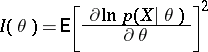is not zero, the Cramér–Rao inequality(1)

holds. This inequality gives a lower bound for the mean-square error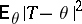of all estimatorsfor the unknown parameterthat have the same bias function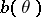.

In particular, ifis an unbiased estimator for, that is, if, then (1) implies that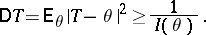(2)

Thus, in this case the Cramér–Rao inequality provides a lower bound for the variance of the unbiased estimatorsfor, equal to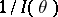, and also demonstrates that the existence of consistent estimators (cf. Consistent estimator) is connected with unrestricted growth of the Fisher information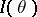as. If equality is attained in (2) for a certain unbiased estimator, thenis optimal in the class of all unbiased estimators with regard to minimum quadratic risk; it is called an efficient estimator. For example, ifare independent random variables subject to the same normal law, then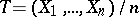is an efficient estimator of the unknown mean.

In general, equality in (2) is attained if and only ifis an exponential family, that is, if the probability density ofcan be represented in the form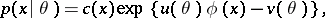in which case the sufficient statistic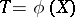is an efficient estimator of its expectation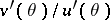. If no efficient estimator exists, the lower bound of the variances of the unbiased estimators can be refined, since the Cramér–Rao inequality does not necessarily give the greatest lower bound. For example, ifare independent random variables with the same normal distribution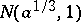, then the greatest lower bound to the variance of unbiased estimators ofis equal to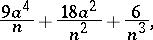whileIn general, absence of equality in (2) does not mean that the estimator that has been found is not optimal, since it may well be the only unbiased estimator.

There are different generalizations of the Cramér–Rao inequality, to the case of a vector parameter, or to that of estimating a function of the parameter. Refinements of the lower bound in (2) play an important role in such cases.

The inequality (1) was independently obtained by M. Fréchet, C.R. Rao and H. Cramér.

How to Cite This Entry:
Rao-Cramér inequality. Encyclopedia of Mathematics. URL: http://encyclopediaofmath.org/index.php?title=Rao-Cram%C3%A9r_inequality&oldid=13110
This article was adapted from an original article by M.S. Nikulin (originator), which appeared in Encyclopedia of Mathematics - ISBN 1402006098. See original article